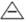Chapter 8.1, Problem 22ESMathematical Excursions (MindTap C...

4th Edition
Richard N. Aufmann + 3 others
ISBN: 9781305965584

Solutions

Chapter
SectionMathematical Excursions (MindTap C...

4th Edition
Richard N. Aufmann + 3 others
ISBN: 9781305965584
Textbook Problem

Military Time. Evaluate each expression, where + and − indicate addition and subtraction, respectively, using military time. (Military time uses a 24-hour clock, where 2:00 A.M. is equivalent to 0200 hours and 10 P.M. is equivalent to 2200 hours.) 1800 − 1900

To determine

The addition 18001900 using military time.

Explanation

Given information: denote the addition on 24-hour clock military time.

In military time, 2:00 A. M. is equivalent to 0200 hours and 10:00 P. M. is equivalent to 2200 hours.

Calculation:

1800

Still sussing out bartleby?

Check out a sample textbook solution.

See a sample solution

The Solution to Your Study Problems

Bartleby provides explanations to thousands of textbook problems written by our experts, many with advanced degrees!

Get Started